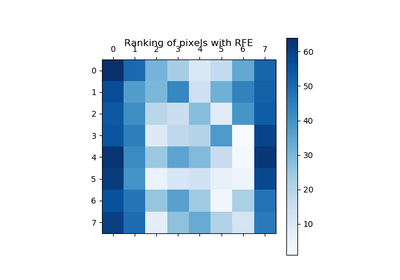# `sklearn.feature_selection`.RFE¶

class `sklearn.feature_selection.``RFE`(estimator, n_features_to_select=None, step=1, verbose=0)[source]

Feature ranking with recursive feature elimination.

Given an external estimator that assigns weights to features (e.g., the coefficients of a linear model), the goal of recursive feature elimination (RFE) is to select features by recursively considering smaller and smaller sets of features. First, the estimator is trained on the initial set of features and the importance of each feature is obtained either through a `coef_` attribute or through a `feature_importances_` attribute. Then, the least important features are pruned from current set of features. That procedure is recursively repeated on the pruned set until the desired number of features to select is eventually reached.

Read more in the User Guide.

Parameters: estimator : object A supervised learning estimator with a `fit` method that provides information about feature importance either through a `coef_` attribute or through a `feature_importances_` attribute. n_features_to_select : int or None (default=None) The number of features to select. If None, half of the features are selected. step : int or float, optional (default=1) If greater than or equal to 1, then step corresponds to the (integer) number of features to remove at each iteration. If within (0.0, 1.0), then step corresponds to the percentage (rounded down) of features to remove at each iteration. verbose : int, default=0 Controls verbosity of output. n_features_ : int The number of selected features. support_ : array of shape [n_features] The mask of selected features. ranking_ : array of shape [n_features] The feature ranking, such that `ranking_[i]` corresponds to the ranking position of the i-th feature. Selected (i.e., estimated best) features are assigned rank 1. estimator_ : object The external estimator fit on the reduced dataset.

References

 [R170] Guyon, I., Weston, J., Barnhill, S., & Vapnik, V., “Gene selection for cancer classification using support vector machines”, Mach. Learn., 46(1-3), 389–422, 2002.

Examples

The following example shows how to retrieve the 5 right informative features in the Friedman #1 dataset.

```>>> from sklearn.datasets import make_friedman1
>>> from sklearn.feature_selection import RFE
>>> from sklearn.svm import SVR
>>> X, y = make_friedman1(n_samples=50, n_features=10, random_state=0)
>>> estimator = SVR(kernel="linear")
>>> selector = RFE(estimator, 5, step=1)
>>> selector = selector.fit(X, y)
>>> selector.support_
array([ True,  True,  True,  True,  True,
False, False, False, False, False], dtype=bool)
>>> selector.ranking_
array([1, 1, 1, 1, 1, 6, 4, 3, 2, 5])
```

Methods

 `decision_function`(X) `fit`(X, y) Fit the RFE model and then the underlying estimator on the selected features. `fit_transform`(X[, y]) Fit to data, then transform it. `get_params`([deep]) Get parameters for this estimator. `get_support`([indices]) Get a mask, or integer index, of the features selected `inverse_transform`(X) Reverse the transformation operation `predict`(X) Reduce X to the selected features and then predict using the underlying estimator. `predict_log_proba`(X) `predict_proba`(X) `score`(X, y) Reduce X to the selected features and then return the score of the underlying estimator. `set_params`(**params) Set the parameters of this estimator. `transform`(X) Reduce X to the selected features.
`__init__`(estimator, n_features_to_select=None, step=1, verbose=0)[source]
`fit`(X, y)[source]
Fit the RFE model and then the underlying estimator on the selected
features.
Parameters: X : {array-like, sparse matrix}, shape = [n_samples, n_features] The training input samples. y : array-like, shape = [n_samples] The target values.
`fit_transform`(X, y=None, **fit_params)[source]

Fit to data, then transform it.

Fits transformer to X and y with optional parameters fit_params and returns a transformed version of X.

Parameters: X : numpy array of shape [n_samples, n_features] Training set. y : numpy array of shape [n_samples] Target values. X_new : numpy array of shape [n_samples, n_features_new] Transformed array.
`get_params`(deep=True)[source]

Get parameters for this estimator.

Parameters: deep : boolean, optional If True, will return the parameters for this estimator and contained subobjects that are estimators. params : mapping of string to any Parameter names mapped to their values.
`get_support`(indices=False)[source]

Get a mask, or integer index, of the features selected

Parameters: indices : boolean (default False) If True, the return value will be an array of integers, rather than a boolean mask. support : array An index that selects the retained features from a feature vector. If indices is False, this is a boolean array of shape [# input features], in which an element is True iff its corresponding feature is selected for retention. If indices is True, this is an integer array of shape [# output features] whose values are indices into the input feature vector.
`inverse_transform`(X)[source]

Reverse the transformation operation

Parameters: X : array of shape [n_samples, n_selected_features] The input samples. X_r : array of shape [n_samples, n_original_features] X with columns of zeros inserted where features would have been removed by transform.
`predict`(X)[source]
Reduce X to the selected features and then predict using the
underlying estimator.
Parameters: X : array of shape [n_samples, n_features] The input samples. y : array of shape [n_samples] The predicted target values.
`score`(X, y)[source]
Reduce X to the selected features and then return the score of the
underlying estimator.
Parameters: X : array of shape [n_samples, n_features] The input samples. y : array of shape [n_samples] The target values.
`set_params`(**params)[source]

Set the parameters of this estimator.

The method works on simple estimators as well as on nested objects (such as pipelines). The latter have parameters of the form `<component>__<parameter>` so that it’s possible to update each component of a nested object.

Returns: self :
`transform`(X)[source]

Reduce X to the selected features.

Parameters: X : array of shape [n_samples, n_features] The input samples. X_r : array of shape [n_samples, n_selected_features] The input samples with only the selected features.

## Examples using `sklearn.feature_selection.RFE`¶Recursive feature elimination